机器学习笔记之（4）——Fisher分类器（线性判别分析，LDA）

11 篇文章 11 订阅

• 《Python大战机器学习》

Fisher分类器也叫Fisher线性判别（Fisher Linear Discriminant），或称为线性判别分析（Linear Discriminant Analysis，LDA）。LDA有时也被称为Fisher's LDA。最初于1936年，提出Fisher线性判别，后来于1948年，进行改进成如今所说的LDA。

线性模型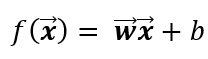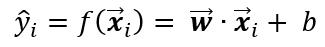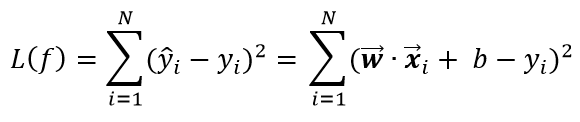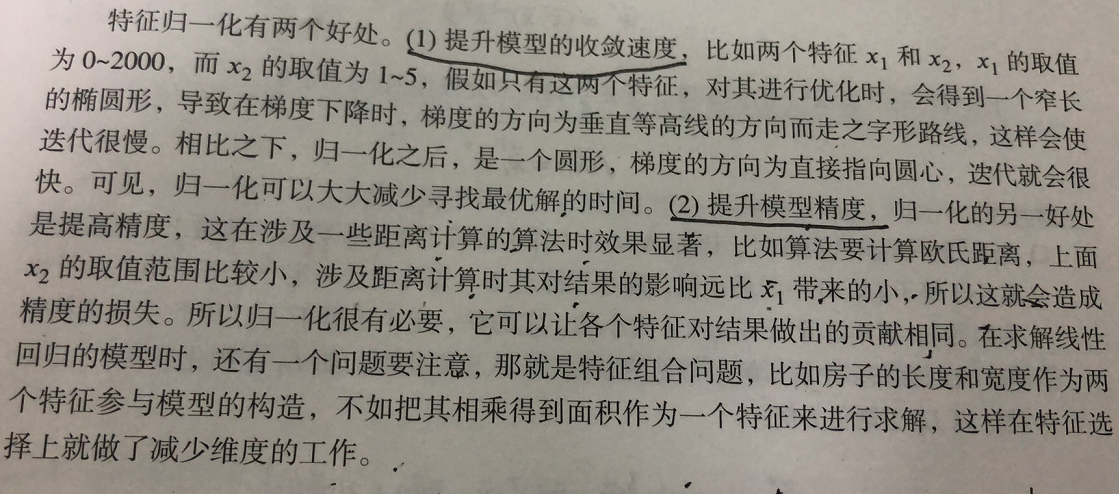LDA或Fisher：

Fisher的基本思想是：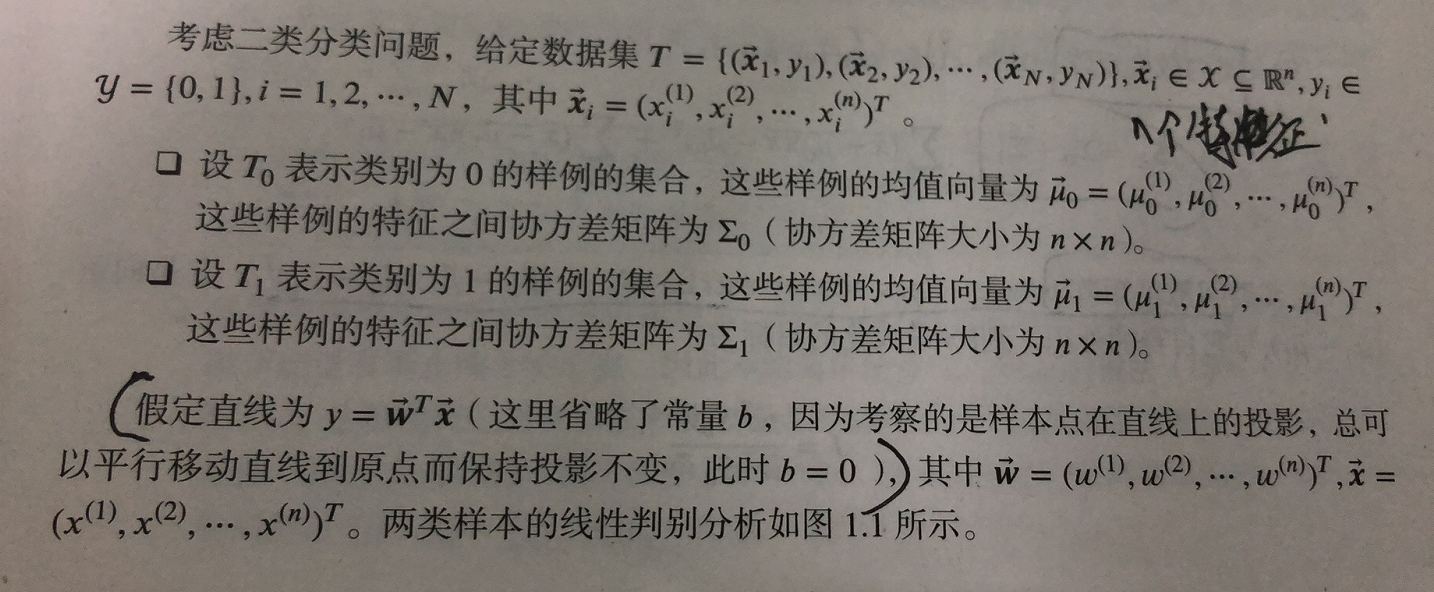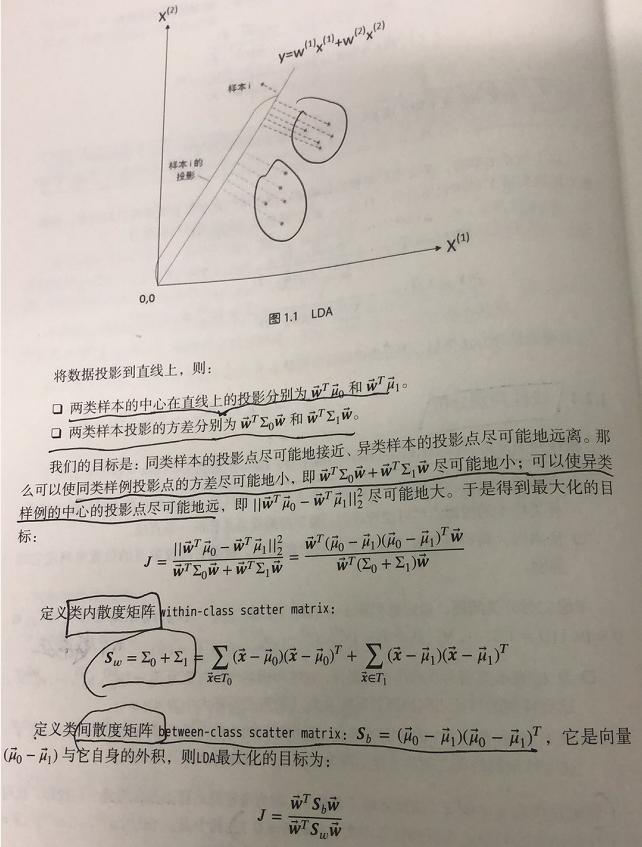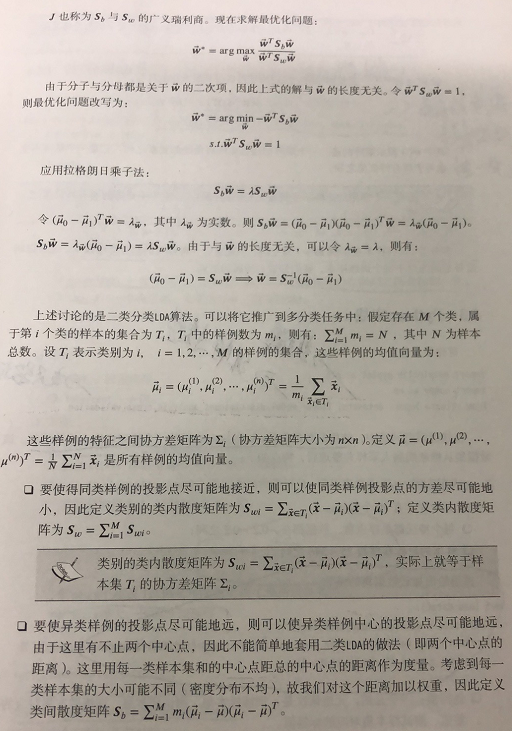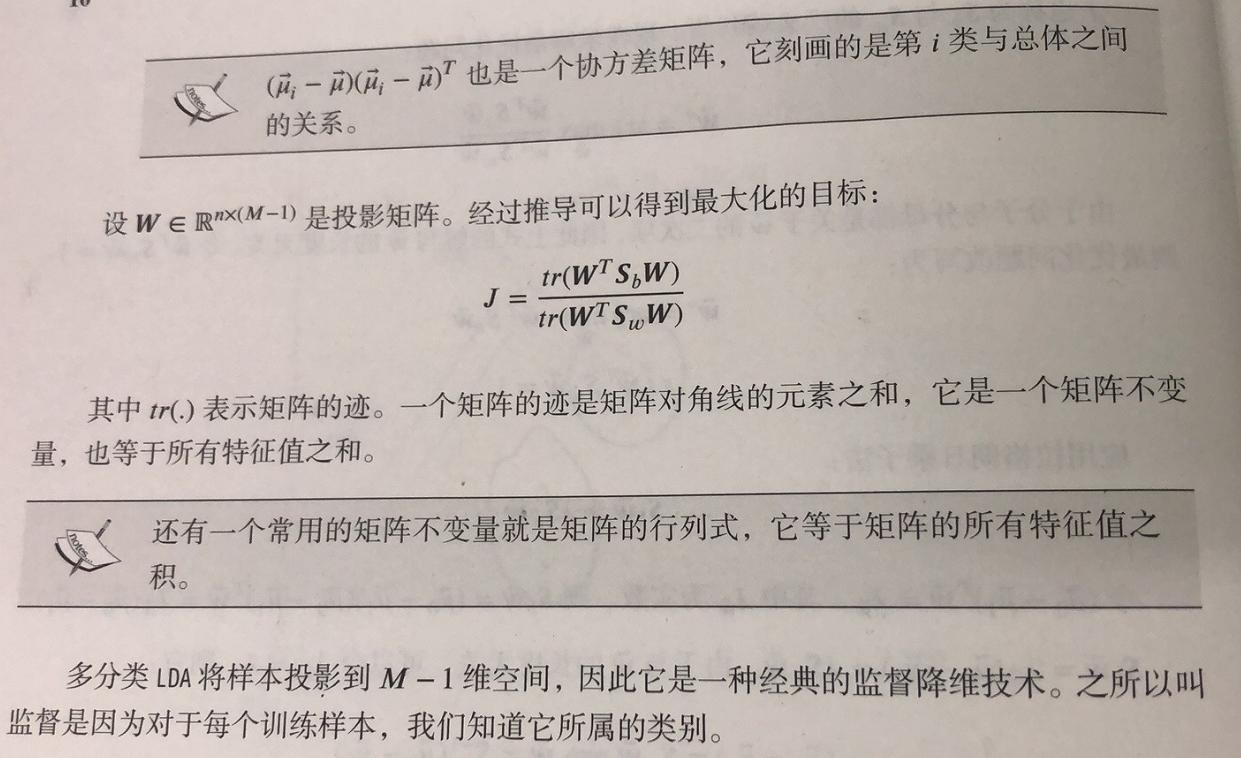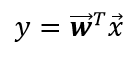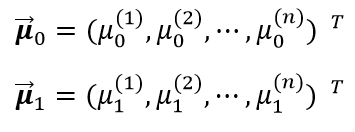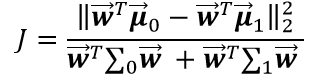给出Python代码如下：

from sklearn import datasets, cross_validation,discriminant_analysis

###############################################################################
#用莺尾花数据集
return cross_validation.train_test_split(iris.data,iris.target,test_size=0.25,random_state=0,stratify=iris.target)
#返回为: 一个元组，依次为：训练样本集、测试样本集、训练样本的标记、测试样本的标记

###############################################################################
def test_LinearDiscriminantAnalysis(*data):
x_train,x_test,y_train,y_test=data
lda=discriminant_analysis.LinearDiscriminantAnalysis()
lda.fit(x_train,y_train)
print('Coefficients:%s, intercept %s'%(lda.coef_,lda.intercept_))#输出权重向量和b
print('Score: %.2f' % lda.score(x_test, y_test))#测试集
print('Score: %.2f' % lda.score(x_train, y_train))#训练集

###############################################################################
test_LinearDiscriminantAnalysis(x_train,x_test,y_train,y_test)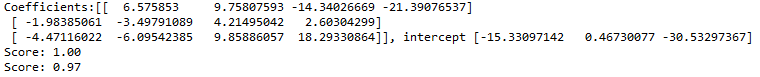Fisher的这种映射关系还有一种作用就是作为降维技术，称为监督降维技术（因为是有训练数据的，所以称为监督）

from sklearn import datasets, cross_validation,discriminant_analysis

###############################################################################
#用莺尾花数据集
return cross_validation.train_test_split(iris.data,iris.target,test_size=0.25,random_state=0,stratify=iris.target)
#返回为: 一个元组，依次为：训练样本集、测试样本集、训练样本的标记、测试样本的标记

###############################################################################
def plot_LDA(converted_X,y):
'''
绘制经过 LDA 转换后的数据

:param converted_X: 经过 LDA转换后的样本集
:param y: 样本集的标记
:return:  None
'''
from mpl_toolkits.mplot3d import Axes3D
import matplotlib.pyplot as plt
fig=plt.figure()
ax=Axes3D(fig)
colors='rgb'
markers='o*s'
for target,color,marker in zip([0,1,2],colors,markers):
pos=(y==target).ravel()
X=converted_X[pos,:]
ax.scatter(X[:,0], X[:,1], X[:,2],color=color,marker=marker,
label="Label %d"%target)
ax.legend(loc="best")
fig.suptitle("Iris After LDA")
plt.show()

###############################################################################
import numpy as np
X=np.vstack((x_train,x_test))#沿着竖直方向将矩阵堆叠起来，把训练与测试的数据放一起来看
Y=np.vstack((y_train.reshape(y_train.size,1),y_test.reshape(y_test.size,1)))#沿着竖直方向将矩阵堆叠起来
lda = discriminant_analysis.LinearDiscriminantAnalysis()
lda.fit(X, Y)
converted_X=np.dot(X,np.transpose(lda.coef_))+lda.intercept_
plot_LDA(converted_X,Y)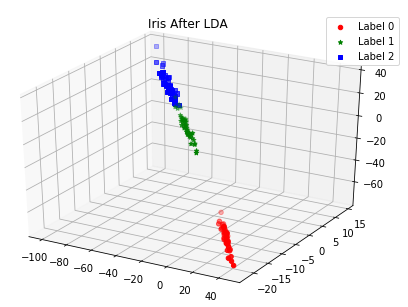补充：

LDA是一种可作为特征抽取的技术，它可以提高数据分析过程中的计算效率，同时，对于不适用于正则化的模型，它可以降低因维度灾难带来的过拟合。

LDA的基本概念与PCA非常相似（见博文机器学习笔记6）。PCA试图在数据集中找到方差最大的正交的主成分分量的轴，而LDA的目标是发现可以最优化分类的特征子空间。其中，PCA是无监督算法，而LDA是监督算法，因此，直观上，LDA比PCA更优越。如下图所示：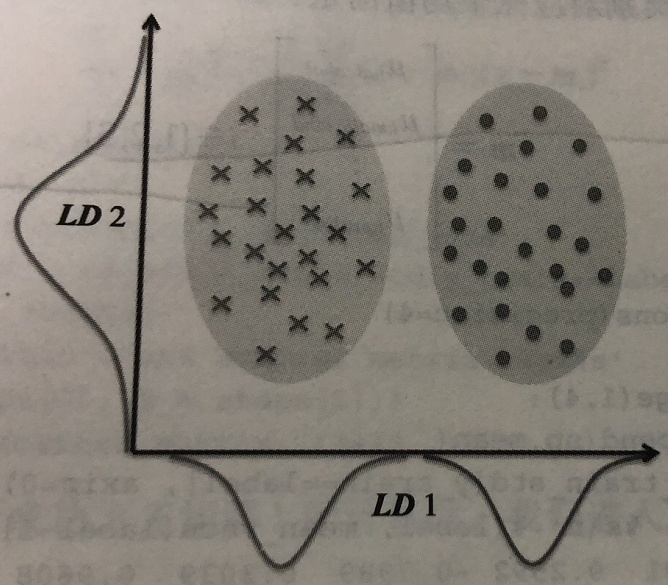#####################################定义一个画图函数###########################
import numpy as np
import matplotlib.pyplot as plt
from matplotlib.colors import ListedColormap

def plot_decision_regions(X, y, classifier, resolution=0.02):

# setup marker generator and color map
markers = ('s', 'x', 'o', '^', 'v')
colors = ('red', 'blue', 'lightgreen', 'gray', 'cyan')
cmap = ListedColormap(colors[:len(np.unique(y))])

# plot the decision surface
x1_min, x1_max = X[:, 0].min() - 1, X[:, 0].max() + 1
x2_min, x2_max = X[:, 1].min() - 1, X[:, 1].max() + 1
xx1, xx2 = np.meshgrid(np.arange(x1_min, x1_max, resolution),
np.arange(x2_min, x2_max, resolution))
Z = classifier.predict(np.array([xx1.ravel(), xx2.ravel()]).T)
Z = Z.reshape(xx1.shape)
plt.contourf(xx1, xx2, Z, alpha=0.4, cmap=cmap)
plt.xlim(xx1.min(), xx1.max())
plt.ylim(xx2.min(), xx2.max())

# plot class samples
for idx, cl in enumerate(np.unique(y)):
plt.scatter(x=X[y == cl, 0],
y=X[y == cl, 1],
alpha=0.6,
c=cmap(idx),
edgecolor='black',
marker=markers[idx],
label=cl)

##############################数据的读入、划分、标准化###########################
import pandas as pd
#Python Data Analysis Library 或 pandas 是基于NumPy 的一种工具，该工具是为了解决数据分析任务而创建的。
#Pandas 纳入了大量库和一些标准的数据模型，提供了高效地操作大型数据集所需的工具。
#读入csv文件，形成一个数据框
#使用葡萄酒数据集

from sklearn.cross_validation import train_test_split
x, y = df_wine.iloc[:, 1:].values, df_wine.iloc[:, 0].values
x_train, x_test, y_train, y_test =train_test_split(x, y, test_size=0.3,stratify=y,random_state=0)
#葡萄酒数据集划分为训练集和测试集，分别占据数据集的70%和30%

#使用单位方差对数据集进行标准化
from sklearn.preprocessing import StandardScaler
sc=StandardScaler()
#注意，当改为from sklearn.preprocessing import StandardScaler as sc时，会报错
# fit_transform() missing 1 required positional argument: 'X'。这就是由于sc没有StandardScaler()而仅仅是StandardScaler
x_train_std=sc.fit_transform(x_train)
x_test_std=sc.fit_transform(x_test)

#####################################使用sklearn中的LDA类#######################
from sklearn.linear_model import LogisticRegression
from sklearn.discriminant_analysis import LinearDiscriminantAnalysis as LDA
lda = LDA(n_components=2)#选取两个主成分
#做降维处理
x_train_lda = lda.fit_transform(x_train_std, y_train)
x_test_lda=lda.transform(x_test_std)

#用逻辑斯蒂回归进行分类(对训练数据进行处理)
lr=LogisticRegression()
lr.fit(x_train_lda,y_train)
plot_decision_regions(x_train_lda,y_train,classifier=lr)
plt.xlabel('LD 1')
plt.ylabel('LD 2')
plt.legend(loc='lower left')
plt.tight_layout()
plt.show()

#用逻辑斯蒂回归进行分类(对测试数据进行处理)
lr=LogisticRegression()
lr.fit(x_train_lda,y_train)
plot_decision_regions(x_test_lda,y_test,classifier=lr)
plt.xlabel('LD 1')
plt.ylabel('LD 2')
plt.legend(loc='lower left')
plt.tight_layout()
plt.show()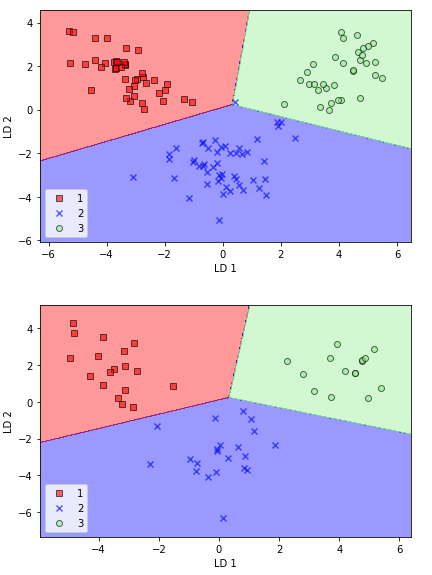• 《Python机器学习》

01-27
12-2601-183万+
04-168214
11-30750
10-141万+
05-192万+
04-163万+
10-242万+
06-281万+
01-197671
12-26
02-22
11-27
12-282353
05-053069
10-28128
11-26236
12-231721
12-112578gwpscut

¥2 ¥4 ¥6 ¥10 ¥20余额支付 (余额：-- )扫码支付获取中扫码支付点击重新获取扫码支付1.余额是钱包充值的虚拟货币，按照1:1的比例进行支付金额的抵扣。
2.余额无法直接购买下载，可以购买VIP、C币套餐、付费专栏及课程。余额充值